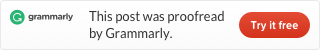# How to calculate ISO week number in Power QueryI calculated ISO week number in Power Query following instructions from Wikipedia.
Here is how it goes. M code is at the end of this post.It all starts with weekday calculation. By default Power Query Date.DayOfWeek function returns a number between 0 and 6 starting from Sunday. To return weekday number according to ISO8601 rules, you have to add optional first-weekday value and change numbering from 1 to 7. For this demonstration, I also generated a list of dates.`=Date.DayOfWeek([Date], Day.Monday)+1`

After this little adjustment, it is possible to calculate ISO week number in Power Query with all necessary conditions.

Using ISO weekday numbers (running from 1 for Monday to 7 for Sunday), subtract the weekday from the ordinal date, then add 10. Divide the result by 7. Ignore the remainder; the quotient equals the week number. If the week number thus obtained equals 0, it means that the given date belongs to the preceding (week-based) year. If a week number of 53 is obtained, one must check that the date is not actually in week 1 of the following year.

```=if
Number.RoundDown((Date.DayOfYear([Date])-(Date.DayOfWeek([Date], Day.Monday)+1)+10)/7)=0

then
Number.RoundDown((Date.DayOfYear(#date(Date.Year([Date])-1,12,31))-(Date.DayOfWeek(#date(Date.Year([Date])-1,12,31), Day.Monday)+1)+10)/7)

else if
(Number.RoundDown((Date.DayOfYear([Date])-(Date.DayOfWeek([Date], Day.Monday)+1)+10)/7)=53
and (Date.DayOfWeek(#date(Date.Year([Date]),12,31), Day.Monday)+1<4))

then
1

else
Number.RoundDown((Date.DayOfYear([Date])-(Date.DayOfWeek([Date], Day.Monday)+1)+10)/7)```

Here is M code that you can put in Blank Query and try by yourself.

```//datacornering.com

let
Source = List.Dates(DateTime.Date(DateTime.LocalNow()), 365, #duration(1, 0, 0, 0)),
#"Converted to Table" = Table.FromList(Source, Splitter.SplitByNothing(), null, null, ExtraValues.Error),
#"Renamed Columns" = Table.RenameColumns(#"Converted to Table",{{"Column1", "Date"}}),
#"Changed Type" = Table.TransformColumnTypes(#"Renamed Columns",{{"Date", type date}}),
#"Weekday Number" = Table.AddColumn(#"Changed Type", "Weekday Number", each Date.DayOfWeek([Date], Day.Monday)+1),

#"ISO Week Number" = Table.AddColumn(#"Weekday Number", "ISO Week Number", each if
Number.RoundDown((Date.DayOfYear([Date])-(Date.DayOfWeek([Date], Day.Monday)+1)+10)/7)=0

then
Number.RoundDown((Date.DayOfYear(#date(Date.Year([Date])-1,12,31))-(Date.DayOfWeek(#date(Date.Year([Date])-1,12,31), Day.Monday)+1)+10)/7)

else if
(Number.RoundDown((Date.DayOfYear([Date])-(Date.DayOfWeek([Date], Day.Monday)+1)+10)/7)=53
and (Date.DayOfWeek(#date(Date.Year([Date]),12,31), Day.Monday)+1<4))

then
1

else
Number.RoundDown((Date.DayOfYear([Date])-(Date.DayOfWeek([Date], Day.Monday)+1)+10)/7))

in
#"ISO Week Number"```

### 16 comments on “How to calculate ISO week number in Power Query”

1.Carina Lindebjerg Larsen

This is just what I need! Can you also show how to add the proper year? based on the IOS column?

•Janis Sturis

What do you mean with “proper year”? Please give an example.

•Robert Maes

He means this:

Imagine a column like “Year – Weeknumber” > “2020 – 53”.
In 2021, the 1st of July fell on a Friday. This means that the 1st, 2nd and 3rd of January belong to week 53 of the previous year. These dates, from 2021, should thus fall under “2020 – 53”.
Consequently, the week of 04-01-2021 to 10-01-2021 is “2021 – 01”

•Bruce Diepgrond

I looked it up, and every in my region (Europe) the first week of the year is the week with at least 4 days in the new year, as Robert explained (think the 1st of july was a type-o and should be 1st of january). Also our weeks start on a Monday, in some regions it sunday.

If you calculated the ISO week and had put it in a column [Week]
and calculated the Year and put it in a Column [Year]
and have the column with the Date [Date]
you can Calculate the ISO Year as follows:

DAX:
Iso Year = IF([Week]>51 && DAY([Date])<4,[Year]-1,IF([Week]28,[Year]+1, [Year]))

M:
if ([Week]>51 and Date.Day([Date])<4) then [Year]-1 else if ([Week]28) then [Year]+1 else [Year]

DAY([Date]) and Date.Day([Date]) tells you the day number of the month (1-31)

Hope this helps.

•Bruce Diepgrond

Sorry, I see i pasted my M code wrongly

if ([Week]>51 and Date.Day([Date])<4) then [Year]-1 else if ([Week]28) then [Year]+1 else [Year]

•Janis Sturis

Thank you for your answer!

•Rob Light

Thank you for this Bruce, really helpful. Can you explain what the ‘else if ([Week]28)’ refers to? I can’t get it to work properly.

•Prateeksha

Hi Bruce,

Is there supposed to be a symbol before the 28?

Your help is greatly appreciated

2.zedleb

Brilliant! Thank you so much Janis.

3.Sergio

hi,
I think you forgot a sign on: if ([Week]28)…

•Khoi Pham

Great!

if ([Week]>51 and Date.Day([Date])51 and Date.Day([Date])<4) then [Year]-1 else [Year]

What are the limitations of not using the week28 comparison.

4.Prateeksha

Hi Khoi,
Is there a sign that should be before the 51?

5.Ken Edwards

For 2021 I have the first 3 days showing up as week 53. How do I modify the code to have these first 3 days show as week 1

6.Manasi

Hello

I do have same error of 53, weeks showing of first day of Feb as 53.

I used above query, but its showing week 5 and 6.

Please help.

•Janis Sturis

Try to use fuction Date.WeekOfYear function https://docs.microsoft.com/en-us/powerquery-m/date-weekofyear